Next: Linear Stark Effect Up: Time-Independent Perturbation Theory Previous: Quadratic Stark Effect

# Degenerate Perturbation Theory

Let us, rather naively, investigate the Stark effect in an excited (i.e.,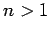) state of the hydrogen atom using standard non-degenerate perturbation theory. We can write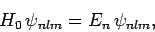(940)

since the energy eigenstates of the unperturbed Hamiltonian only depend on the quantum number. Making use of the selection rules (917) and (927), non-degenerate perturbation theory yields the following expressions for the perturbed energy levels and eigenstates [see Eqs. (909) and (910)]: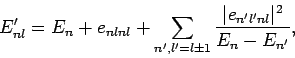(941)

and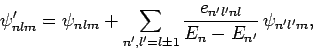(942)

where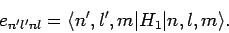(943)

Unfortunately, ifthen the summations in the above expressions are not well-defined, because there exist non-zero matrix elements,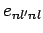, which couple degenerate eigenstates: i.e., there exist non-zero matrix elements which couple states with the same value of, but different values of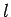. These particular matrix elements give rise to singular factors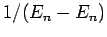in the summations. This does not occur ifbecause, in this case, the selection rule, and the fact that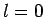(since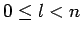), only allow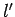to take the single value 1. Of course, there is nostate with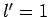. Hence, there is only one coupled state corresponding to the eigenvalue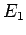. Unfortunately, ifthen there are multiple coupled states corresponding to the eigenvalue.

Note that our problem would disappear if the matrix elements of the perturbed Hamiltonian corresponding to the same value of, but different values of, were all zero: i.e., if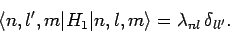(944)

In this case, all of the singular terms in Eqs. (941) and (942) would reduce to zero. Unfortunately, the above equation is not satisfied. Fortunately, we can always redefine the unperturbed eigenstates corresponding to the eigenvaluein such a manner that Eq. (944) is satisfied. Suppose that there are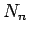coupled eigenstates belonging to the eigenvalue. Let us definenew states which are linear combinations of ouroriginal degenerate eigenstates: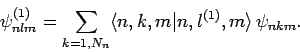(945)

Note that these new states are also degenerate energy eigenstates of the unperturbed Hamiltonian,, corresponding to the eigenvalue. The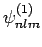are chosen in such a manner that they are also eigenstates of the perturbing Hamiltonian,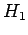: i.e., they are simultaneous eigenstates ofand. Thus,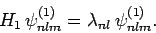(946)

Theare also chosen so as to be orthonormal: i.e.,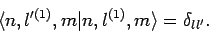(947)

It follows that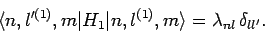(948)

Thus, if we use the new eigenstates, instead of the old ones, then we can employ Eqs. (941) and (942) directly, since all of the singular terms vanish. The only remaining difficulty is to determine the new eigenstates in terms of the original ones.

Now [see Eq. (874)]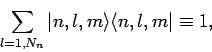(949)

wheredenotes the identity operator in the sub-space of all coupled unperturbed eigenstates corresponding to the eigenvalue. Using this completeness relation, the eigenvalue equation (946) can be transformed into a straightforward matrix equation: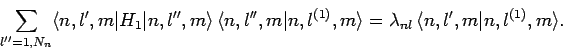(950)

This can be written more transparently as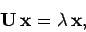(951)

where the elements of the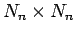Hermitian matrix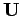are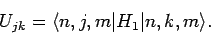(952)

Provided that the determinant ofis non-zero, Eq. (951) can always be solved to giveeigenvalues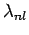(for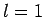to), withcorresponding eigenvectors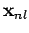. The normalized eigenvectors specify the weights of the new eigenstates in terms of the original eigenstates: i.e.,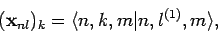(953)

for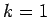to. In our new scheme, Eqs. (941) and (942) yield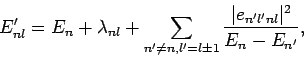(954)

and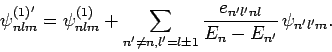(955)

There are no singular terms in these expressions, since the summations are over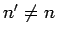: i.e., they specifically exclude the problematic, degenerate, unperturbed energy eigenstates corresponding to the eigenvalue. Note that the first-order energy shifts are equivalent to the eigenvalues of the matrix equation (951).Next: Linear Stark Effect Up: Time-Independent Perturbation Theory Previous: Quadratic Stark Effect
Richard Fitzpatrick 2010-07-20